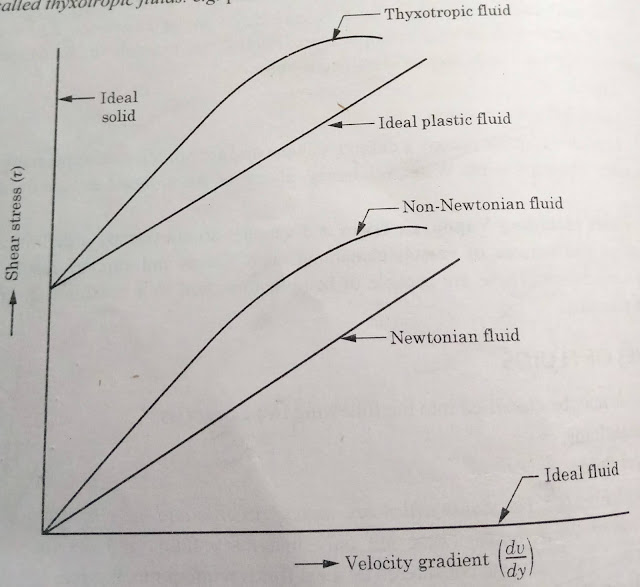# Basic Concepts of Fluid Mechanics | QnA | Fluid Mechanics | By Ashutosh Nautiyal

Definition of fluid. or What is fluid? or Define fluid. or Describe fluid? The fluid is defined as any substance which is capable of flowing.

## Basic Concepts of Fluid MechanicsIntroduction and Basic Concepts of Fluid Mechanics

## Describe fluid.

• The fluid is defined as any substance which is capable of flowing and offers practically no resistance to the change of its shape or which deforms continuously under the effect of external shearing force.

• Literally, it means anything which can flow but technically it includes liquids and gases.

## How Fluid is different from Solid?

• The solid can resist the shear force or shear stress under static equilibrium condition with the finite deformation and it can also regain partly or wholly its original shape when the load is relieved.

• But, in the case of fluid, the fluid goes on continuously deforming which means that fluid resists tangential force only under the dynamic condition not under the static condition and all fluids (except visco-elastic fluid) cannot regain or partly regain their original shape.

## Ideal Fluids

• The fluids which are incompressible and have no viscosity and surface tension are called Ideal Fluids.

• These are imaginary fluids.

• They do not exist in nature.

## Real Fluids

• The fluids which possess properties such as viscosity, surface tension and compressibility are called Real Fluids.

• The fluids actually available in nature are Real Fluids.

• These fluids offer a certain amount of resistance when these are set in motion.

## Describe the different types of Real Fluids.Types of Real Fluids

## Newtonian Fluids

• The fluids in which shear stress is directly proportional to the rate of shear strain (or velocity gradient) are called Newtonian Fluids.

• These fluids follow Newton's Law of Viscosity.

## Non-Newtonian Fluids

• The fluids in which shear stress is not proportional to the rate of shear strain (or velocity gradient) are called Non-Newtonian Fluids.

## Ideal Plastic Fluids

• The fluids in which shear stress is more than yield stress value and shear stress is directly proportional to the rate of shear strain (or velocity gradient) are called Ideal Plastic Fluids.

## Thixotropic or Thyxotropic Fluids

• The fluids in which shear stress is more than yield stress value and the shear stress is not directly proportional to the rate of shear strain (or velocity gradient) are called Thixotropic Fluids.

• Printer's ink is an example of Thixotropic Fluids.

## Describe Fluid Mechanics.

• Fluid Mechanics is the branch of science which deals with the behaviour of fluids (liquids or gases) at rest as well as in motion.

• It is a subject which describes all the physical laws that govern the flow of fluids.

• It also helps us to recognize the causes and effects of flow through the determination of characteristic parameters like pressure field, velocity field in a fluid flow, along with the different properties of the fluid, like density, viscosity, etc.

• Importance of Fluid Mechanics can be seen in the breathing process, circulation of blood, the pressure exerted by the air on us, landing of aircraft, etc.

## Fluid Statics

• The study of fluids at rest is called Fluid Statics.

## Fluid Kinematics

• The study of fluids in motion, when pressure forces are not considered, is called Fluid Kinematics.

## Fluid Dynamics

• The study of fluids in motion, when pressure forces are considered, is called Fluid Dynamics.

*Self Typed
*Source- Books, Internet, Self-Analysis

Getting Info...